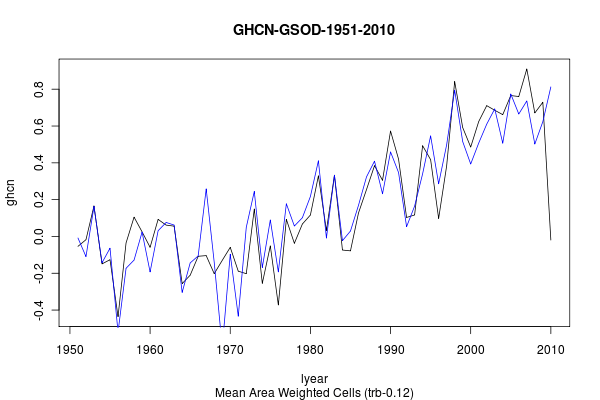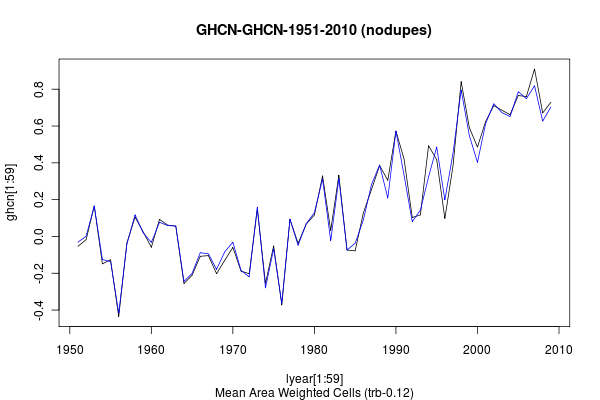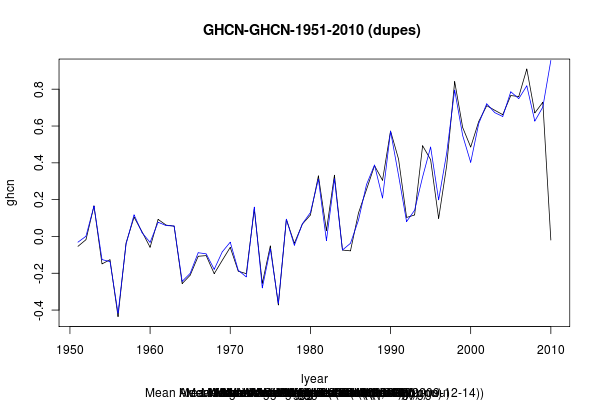Home > GHCN, GSOD, trb > UPDATED: trb-0.12: Gridded Anomalies

## UPDATED: trb-0.12: Gridded Anomalies

2010 August 6

Introduction

Anomalies!
Anemones!src: http://darcywriter.com/SandandSea.html

Notes

I added the anomalization to trb-0.11, fed in GHCN, and was puzzled by the results (see GHCN results below). The anomalization code initially discarded records with a dupe flag greater than 0. I also tried treating each dupe as a separate record. The second was mildy better, but still an obvious ‘miss’ when matched to GHCN or GISTEMP. It also took about 2 hours to run. I spent some time trying to find a way to anomalize the entire data set without looping through the stations but did not find the trick. I might be able to extend the approach I took with the station dupe code in trb-0.13 to improve this part of the code.

While beating on the code, I decided to split the code into modules to make it more readable and extendable. This refactor was labeled trb-0.12.

I discovered that the GSOD data actually mapped pretty well to the GISTEMP data (with an offset). So I added several other weighting methods to trb-0.12: simple mean of cells, unweighted mean of lats, weighted mean of lats, weighted mean of cells. The third method gave the best match to GISTEMP. The fourth method to GHCN. (The GHCN data is actually the data as processed by Zeke’s code and placed into the common spreadsheet)

I was unable to force GHCN into any semblance of existing surface records with either the ‘no dupes’ or ‘dupes as independent records’ method. That had to wait until I added a combine_dupes function in trb-0.13.

UPDATE: I am not sure what went wrong in the GHCN runs presented here first, but a subsequent run on a different computer produced reasonable results. My experiment of ignoring duplicates and using only the first dupe (records with a dupe flag of “0”) comes reasonably close to the GHCN record as calculated by Zeke. The experiment of using all the dupes as separate records drags the surface anomaly down in latter decades. That’s interesting and I have no idea why at this time.

This is all ‘land only’ but without the use of a ‘land mask.’ The land surface is treated as a global surface.

GSOD anomalies are calculated against a 1975-2004 baseline and then an offset is applied.

Code

The following is the anomalization code.

```  convert_anomaly <- function(x,b1,b2,bmin) {
# cut a list of data over the baseline
maskb2 <- x\$year <= b2
#maskb3 <- x\$dupe == 0

# find the length of years for this stn
blen <- as.matrix(tapply(btrim\$year, btrim\$id, length))
bna <- as.numeric(lapply(tapply(btrim[,4], btrim[,2], is.na),sum))
for (i in 1:11) {
# find the monthly na values
bna <- cbind(bna,as.numeric(lapply(tapply(btrim[,i+4], btrim[,2], is.na),sum)))
}
bnamax <- rowMax(bna)

# index of stations with bmin good years
bidx  (bmin - 1)
bstns <- unique(btrim[bidx,2])
lidx <- btrim[,2] %in% bstns
btrim <- btrim[lidx,]

# Trim to valid stations
bmean <- matrix(NA,ncol=length(unique(btrim[,2])),nrow=12)
for (i in 1:12) {
bmean[i,] <- tapply(btrim[,i+3], btrim[,2], mean, na.rm=T)
}
bmean <- t(bmean)
bmean <- matrix(as.integer(bmean + 0.5), ncol=12)

# Create monthly anoms
# x <- mm                       # hold the whole mm array
midx <- x[,2] %in% bstns      # trim to good stations
x <- x[midx,]                 # ...
for (i in 1:length(bstns)) {
nr <- nrow(x[x[,2]==bstns[i],4:15])
bm <- t(matrix(bmean[i,],ncol=nr, nrow=12))
x[x[,2]==bstns[i],4:15] <- x[x[,2]==bstns[i],4:15] - bm
}

btrim <- NULL
blen <- NULL
bidx <- NULL
bna <- NULL
bnamax <- NULL
bmean <- NULL
bm <- NULL
return(x)
}```

The following are the various weighted global mean functions

```# get mean of all values
get_global_mean_w0 <- function(r1) {
return(mean(getValues(r1,), na.rm=T))
}

# get global mean by mean of the mean of each lat
get_global_mean_w1 <- function(r1) {

rlats <- rep(NA,nrow(r1))

for (r in 1:length(rlats)) {
rlats[r] <- mean(getValues(r1,r), na.rm=T)
}
m = mean(rlats, na.rm=T)

return(m)
}

# get global mean - get weighted mean of each lat
get_global_mean_w2 <- function(r1) {

rlats <- rep(NA,nrow(r1))

# weighted
w1 <- 0
w <- get_grid_weights(nrow(r1),ncol(r1))
for (r in 1:length(rlats)) {
rlats[r] <- mean(getValues(r1,r), na.rm=T) * sum(w[r,])
if (! sum(is.na(getValues(r1,r)))%/%72 ) {
w1 <-  w1 + sum(w[r,])
}
}
m = sum(rlats, na.rm=T)/w1

return(m)
}

# get global mean - weighted by individual cells
get_global_mean_w3 <- function(r1) {

# weighted
lats <- nrow(r1)
long <- ncol(r1)
w1 <- 0
s <- 0
w <- get_grid_weights(nrow(r1),ncol(r1))
for (i in 1:lats) {
c1 <- (i-1)*long +1
c2 <- (i)*long
c3 <- seq(c1:c2)+c1-1
s <- s + sum(cellValues(r1,c3) * w[i,1], na.rm=T)
w1 <- w1 + sum(! is.na(cellValues(r1,c3))) * w[i,1]
}
m = s/w1

return(m)
}

# get global mean - get weighted mean of each lat and ocean weighted
get_global_mean_w4 <- function(r1) {

rlats <- rep(NA,nrow(r1))

# weighted
aw <- get_grid_weights(nrow(r1),ncol(r1))
aw1 <- 0
lw1 <- 0
for (r in 1:length(rlats)) {
rlats[r] <- (mean(getValues(r1,r), na.rm=T) * (sum(aw[r,]))*(lw[r,]))
if (! sum(is.na(getValues(r1,r)))%/%72 ) {
aw1 <-  aw1 + sum(w[r,])
lw1 <- lw1 + (lw[r,])
}
}
# the sum of the means / area weights / land weights  / total land fraction
m = sum(rlats, na.rm=T)/aw1 / lw1 / .29

return(m)
}```

Results

The following were made without dupes (my current GSOD files don’t use them) and a 1975-2004 baseline with offsets applied.The following were made dropping the duplicates and using a 1961-1990 baselineThe following were made with duplicates as separate records and using a 1961-1990 baseline1. 2010 August 6 at 5:43 am

You overshot with the pretty picture this time. I just gazed at the picture and ignored the rest of it.

2. 2010 August 6 at 11:27 am

Discarding all non-zero dupes will give you odd behavior, in a large part because not all dupes cover the same period.

Generally I’ve seen a few different ways to deal with dupes, imods, and wmo_ids in the context of the common anomaly method, all of which produce somewhat similar results:

1) Average dupes into imods, average imods into wmo_ids, calculate anomalies for wmo_ids, average wmo_id anomalies into gridboxes

2) Average dupes into imods, calculate anomalies for imods, average imod anomalies into wmo_ids, average wmo_id anomalies into gridboxes

3) Average dupes into imods, calculate anomalies for imods, average imod anomalies into gridboxes

Choice number two seems ideal for a number of reasons, as duplicates can (generally) be safely averaged, while imods should probably not be. However, having two imods at the same location will overweight that location in the gridbox, so averaging imod anomalies into wmo_ids works better.

3. 2010 August 6 at 12:29 pm

I’d use RSM to combine the duplicates. combining the imods is a bit fuzzier.

4. 2010 August 7 at 7:32 am

I must have had some messed up data/history in my working directories. The earlier GHCN runs I presented looked more like noise. These look like close matches. Dropping the dupes and just taking the mean of the stations in the cell seems to work pretty well without using any station/dupe combination code.

5. 2010 August 7 at 2:23 pm

imods can be miles away from each other.

For duplicate processing Ron I have some R that gives you 3 options

1. average
2 median
3. Mid point of the min and max.

I didnt implement RSM. on the todo list

Much harder. probably be a seperate piece of code.

6. 2010 August 7 at 2:26 pm

Err Zeke, I do #3.

7. 2010 August 7 at 3:05 pm

Re: Steven Mosher

Agreed, you should not average imods together because they are supposed to be wholly different stations. You wouldn’t average New York and Boston before anomalizing.

Re: GHCN duplicates for U.S. stations – I’m starting to find that sometimes different duplicate series are the same station simply with minor rounding differences, and other times, duplicates are wholly different stations from the same “city”.

8. 2010 August 8 at 6:55 am

Sum of squares of difference between GISS and my ghcn based on 4 different methods of calculating the global mean from the gridded data.
> sum((lm0-giss)*(lm0-giss))
 2.065021
> sum((lm1-giss)*(lm1-giss))
 1.160241
> sum((lm2-giss)*(lm2-giss)) # this is the mean of weighted latitudinal means
 0.6210873
> sum((lm3-giss)*(lm3-giss))
 1.282837

Sum of squares of difference between zeke’s GHCN and my ghcn based on 4 different methods of calculating the global mean from the gridded data.
> sum((lm0-ghcn)*(lm0-ghcn))
 0.5166909
> sum((lm1-ghcn)*(lm1-ghcn))
 1.543072
> sum((lm2-ghcn)*(lm2-ghcn))
 1.06022
> sum((lm3-ghcn)*(lm3-ghcn)) # this is the mean of area weighted cells
 0.2165203

———-

So using Zeke’s terminology, here is what trb is doing presently,

4. Discard dupes, calculate anomalies for imods, average imod anomalies into grid boxes.

———-

9. 2010 August 9 at 5:16 pm

By the way, I made an (unannounced) change to my GHCN method recently where I loosened my CAM period restriction somewhat. Instead of requiring stations to have a minimum year prior to 1961 and a maximum year post-1990, I require at least 10 years of data in the 1961-1990 period. This brings my estimates a bit more in-line with other reconstructions: http://i81.photobucket.com/albums/j237/hausfath/Picture27-1.png

Latest GHCN data is here: http://drop.io/0yhqyon/asset/latest-ghcn-data-xls

Also, note that the use of a land-mask results in a non-negligible increase in land temps vis-a-vis not land masking.

10. 2010 August 9 at 7:04 pm

The graphs above require 10 years of data for each month in the baseline (1961-1990)
The do not require a minimum number of months per season or year (yet)

Not much surprise that land-mask increases anomaly. From earlier griddings by you folk, we know that adding SST lowers the anomaly. I would expect small island temps to be dominated by the sea surface temps.

1. No trackbacks yet.